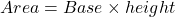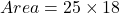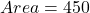## A plot of land is 25 feet in length and 20 feet wide, and it is in the shape of a parallelogram. The perpendicular distance from the long si

Question

A plot of land is 25 feet in length and 20 feet wide, and it is in the shape of a parallelogram. The perpendicular distance from the long side to the opposite long side is 18 feet. What is the area of the piece of land?

in progress 0
3 months 2021-07-30T16:49:27+00:00 1 Answers 2 views 0

1. Given:

Length of the parallelogram = 25 feet

Width of the parallelogram = 20 feet

Perpendicular distance from the long side to the opposite long side = 18 feet.

To find:

The area of the piece of land which is a parallelogram.

Solution:

Area of a parallelogram:Let the longer side, 25 feet is the base, then the height of the parallelogram is 18 feet. So, the area of the parallelogram isTherefore, the area of the piece of land is 450 square feet.